RAOULT'S LAW AND NON-VOLATILE SOLUTES

```
```

This page deals with Raoult's Law and how it applies to solutions in which the solute is non-volatile - for example, a solution of salt in water. A non-volatile solute (the salt, for example) hasn't got any tendency to form a vapour at the temperature of the solution.

It goes on to explain how the resulting lowering of vapour pressure affects the boiling point and freezing point of the solution.

Raoult's Law

There are several ways of stating Raoult's Law, and you tend to use slightly different versions depending on the situation you are talking about. You can use the simplified definition in the box below in the case of a single volatile liquid (the solvent) and a non-volatile solute.

```
```
 The vapour pressure of a solution of a non-volatile solute is equal to the vapour pressure of the pure solvent at that temperature multiplied by its mole fraction.
```
```In this equation, Po is the vapour pressure of the pure solvent at a particular temperature.

xsolv is the mole fraction of the solvent. That is exactly what it says it is - the fraction of the total number of moles present which is solvent.

You calculate this using: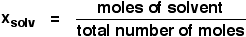Suppose you had a solution containing 10 moles of water and 0.1 moles of sugar. The total number of moles is therefore 10.1

The mole fraction of the water is: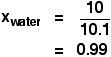```
```

A simple explanation of why Raoult's Law works

There are two ways of explaining why Raoult's Law works - a simple visual way, and a more sophisticated way based on entropy. Because of the level I am aiming at, I'm just going to look at the simple way.

Remember that saturated vapour pressure is what you get when a liquid is in a sealed container. An equilibrium is set up where the number of particles breaking away from the surface is exactly the same as the number sticking on to the surface again.Now suppose you added enough solute so that the solvent molecules only occupied 50% of the surface of the solution.A certain fraction of the solvent molecules will have enough energy to escape from the surface (say, 1 in 1000 or 1 in a million, or whatever). If you reduce the number of solvent molecules on the surface, you are going to reduce the number which can escape in any given time.

But it won't make any difference to the ability of molecules in the vapour to stick to the surface again. If a solvent molecule in the vapour hits a bit of surface occupied by the solute particles, it may well stick. There are obviously attractions between solvent and solute otherwise you wouldn't have a solution in the first place.

The net effect of this is that when equilibrium is established, there will be fewer solvent molecules in the vapour phase - it is less likely that they are going to break away, but there isn't any problem about them returning.

If there are fewer particles in the vapour at equilibrium, the saturated vapour pressure is lower.

```
```

Limitations on Raoult's Law

Raoult's Law only works for ideal solutions. An ideal solution is defined as one which obeys Raoult's Law.

Features of an ideal solution

In practice, there's no such thing! However, very dilute solutions obey Raoult's Law to a reasonable approximation. The solution in the last diagram wouldn't actually obey Raoult's Law - it is far too concentrated. I had to draw it that concentrated to make the point more clearly.

In an ideal solution, it takes exactly the same amount of energy for a solvent molecule to break away from the surface of the solution as it did in the pure solvent. The forces of attraction between solvent and solute are exactly the same as between the original solvent molecules - not a very likely event!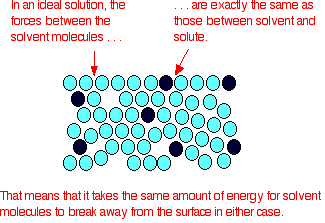Suppose that in the pure solvent, 1 in 1000 molecules had enough energy to overcome the intermolecular forces and break away from the surface in any given time. In an ideal solution, that would still be exactly the same proportion.

Fewer would, of course, break away because there are now fewer solvent molecules on the surface - but of those that are on the surface, the same proportion still break away.

If there were strong solvent-solute attractions, this proportion may be reduced to 1 in 2000, or 1 in 5000 or whatever.

In any real solution of, say, a salt in water, there are strong attractions between the water molecules and the ions. That would tend to slow down the loss of water molecules from the surface. However, if the solution is sufficiently dilute, there will be good-sized regions on the surface where you still have water molecules on their own. The solution will then approach ideal behaviour.

```
```

The nature of the solute

There is another thing that you have to be careful of if you are going to do any calculations on Raoult's Law (beyond the scope of this site). You may have noticed in the little calculation about mole fraction further up the page, that I used sugar as a solute rather than salt. There was a good reason for that!

What matters isn't actually the number of moles of substance that you put into the solution, but the number of moles of particles formed. For each mole of sodium chloride dissolved, you get 1 mole of sodium ions and 1 mole of chloride ions - in other words, you get twice the number of moles of particles as of original salt.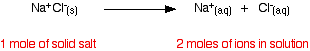So, if you added 0.1 moles of sodium chloride, there would actually be 0.2 moles of particles in the solution - and that's the figure you would have to use in the mole fraction calculation.

Unless you think carefully about it, Raoult's Law only works for solutes which don't change their nature when they dissolve. For example, they mustn't ionise or associate (in other words, if you put in substance A, it mustn't form A2 in solution).

If it does either of these things, you have to treat Raoult's law with great care.

Note:  This isn't a problem you are likely to have to worry about if you are a UK A level student. Just be aware that the problem exists.

Raoult's Law and melting and boiling points

The effect of Raoult's Law is that the saturated vapour pressure of a solution is going to be lower than that of the pure solvent at any particular temperature. That has important effects on the phase diagram of the solvent.

The next diagram shows the phase diagram for pure water in the region around its normal melting and boiling points. The 1 atmosphere line shows the conditions for measuring the normal melting and boiling points.Note:  In common with most phase diagrams, this is drawn highly distorted in order to show more clearly what is going on.

If you haven't already read my page about phase diagrams for pure substances, you should follow this link before you go on to make proper sense of what comes next.

The line separating the liquid and vapour regions is the set of conditions where liquid and vapour are in equilibrium.

It can be thought of as the effect of pressure on the boiling point of the water, but it is also the curve showing the effect of temperature on the saturated vapour pressure of the water. These two ways of looking at the same line are discussed briefly in a note about half-way down the page about phase diagrams (follow the last link above).

If you draw the saturated vapour pressure curve for a solution of a non-volatile solute in water, it will always be lower than the curve for the pure water.Note:  The curves for the pure water and for the solution are often drawn parallel to each other. That has got to be wrong!

Suppose you have a solution where the mole fraction of the water is 0.99 and the vapour pressure of the pure water at that temperature is 100 kPa. The vapour pressure of the solution will be 99 kPa - a fall of 1 kPa. At a lower temperature, where the vapour pressure of the pure water is 10 kPa, the fall will only be 0.1 kPa. For the curves to be parallel the falls would have to be the same over the whole temperature range. They aren't!

If you look closely at the last diagram, you will see that the point at which the liquid-vapour equilibrium curve meets the solid-vapour curve has moved. That point is the triple point of the system - a unique set of temperature and pressure conditions at which it is possible to get solid, liquid and vapour all in equilibrium with each other at the same time.

Since the triple point has solid-liquid equilibrium present (amongst other equilibria), it is also a melting point of the system - although not the normal melting point because the pressure isn't 1 atmosphere.

That must mean that the phase diagram needs a new melting point line (a solid-liquid equilibrium line) passing through the new triple point. That is shown in the next diagram.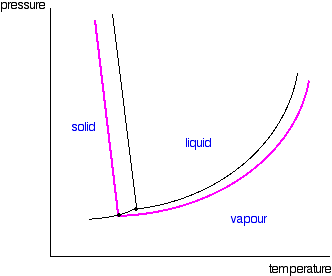Now we are finally in a position to see what effect a non-volatile solute has on the melting and freezing points of the solution. Look at what happens when you draw in the 1 atmosphere pressure line which lets you measure the melting and boiling points. The diagram also includes the melting and boiling points of the pure water from the original phase diagram for pure water (black lines).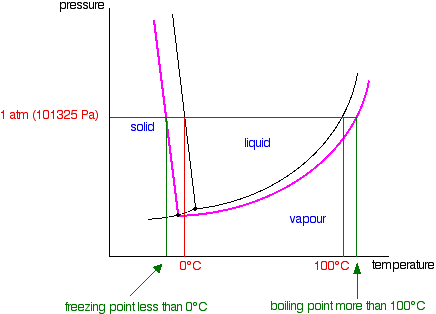```
```

Because of the changes to the phase diagram, you can see that:

• the boiling point of the solvent in a solution is higher than that of the pure solvent;

• the freezing point (melting point) of the solvent in a solution is lower than that of the pure solvent.

```
```

We have looked at this with water as the solvent, but using a different solvent would make no difference to the argument or the conclusions.

The only difference is in the slope of the solid-liquid equilibrium lines. For most solvents, these slope forwards whereas the water line slopes backwards. You could prove to yourself that that doesn't affect what we have been looking at by re-drawing all these diagrams with the slope of that particular line changed.

You will find it makes no difference whatsoever.

```
```
 Questions to test your understanding If this is the first set of questions you have done, please read the introductory page before you start. You will need to use the BACK BUTTON on your browser to come back here afterwards. questions on Raoult's Law and non-volatile solutes answers
```
```

Where would you like to go now?

To the phase equilibrium menu . . .

To the Physical Chemistry menu . . .

To Main Menu . . .

```
```

© Jim Clark 2004 (modified January 2014)